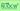# Multiple zeta values and modular formsLecturer Bachmann, Henrik, Associate Professor Graduate School of Mathematics, 2020 Spring Master's student in Graduate School of Mathematics

### Content

Multiple zeta values are real numbers appearing in several areas of mathematics and theoretical physics. These numbers are natural generalizations of special values of the Riemann zeta function. They have been studied at least since Euler, who found many of their algebraic properties. Having been seemingly forgotten for more than 200 years, multiple zeta values were rediscovered by many mathematicians and theoretical physicists since the 1980s in several different contexts (modular forms, mixed Tate motives, quantum groups, moduli spaces of vector bundles, scattering amplitudes, resurgence theory, etc.). (See the textbooks/lecture notes [AK], [BF], [W] and [Z])

### Goals of the Course

The goal of this course is to give an introduction to the theory of multiple zeta values and to explain their connection to modular forms as given in [GKZ] and [B2].

### Topics

1. The Riemann zeta-function and its special values ([AK], ​[BF], [W], [Z])
2. Multiple zeta values and their algebraic structure (harmonic & shuffle product) ([AK], [IKZ], [Z])
3. Regularization of multiple zeta values ([IKZ])
4. (Extended) Double shuffle relations ([IKZ])
5. Families of linear relations among multiple zeta values ([B1], [IKZ], [Zh])
6. Modular forms and their period polynomials ([GKZ], [Za])
7. Connection of modular forms and multiple zeta values ([GKZ])
8. Multiple Eisenstein series ([B2], [GKZ])
9. q-analogues of multiple zeta values ([B2])